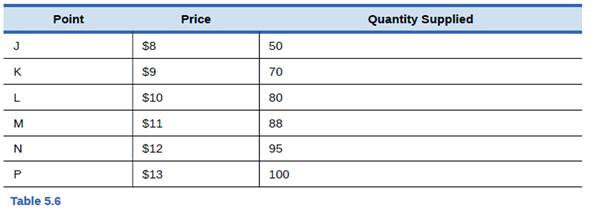Chapter 5, Problem 2SCQ### Principles of Economics 2e

2nd Edition
Steven A. Greenlaw; David Shapiro
ISBN: 9781947172364

#### Solutions

Chapter
Section### Principles of Economics 2e

2nd Edition
Steven A. Greenlaw; David Shapiro
ISBN: 9781947172364
Textbook Problem

# From the data in Table 5.6 about supply of alarm clocks, calculate the price elasticity of supply from: point J to point K, point L to point M, and point N to point P. Classify the elasticity at each point as elastic, inelastic, or unit elastic.To determine

Calculate the elasticity of supply at various points on the supply curve and classify them as inelastic, unit elastic and elastic.

Explanation

Let’s calculate the price elasticity of supply from point J to K:

e =  ΔQ ΔP × P Q

e =  2,6002,800 8070 × 70 2,800

e =  200 10 ×0.025

e = (-) -20 ×0.025

e = 0.5

Therefore, the price elasticity of supply from point J to K is 3.2. Since the elasticity of supply is more than 1, the supply is elastic, which means there is large change in quantity supplied as compared to the change in price level.

Let’s calculate the price elasticity of supply from point L to M:

e =  ΔQ ΔP × P Q

e =  8880 1110 × 10 80

e =  8 1 ×0

### Still sussing out bartleby?

Check out a sample textbook solution.

See a sample solution

#### The Solution to Your Study Problems

Bartleby provides explanations to thousands of textbook problems written by our experts, many with advanced degrees!

Get Started

#### In what ways is economics a science?

Principles of Microeconomics (MindTap Course List)

#### What is normal costing? How does it differ from actual costing?

Managerial Accounting: The Cornerstone of Business Decision-Making

#### Why are convertibles and bonds with warrants typically offered with lower coupons than similarly rated straight...

Fundamentals of Financial Management, Concise Edition (with Thomson ONE - Business School Edition, 1 term (6 months) Printed Access Card) (MindTap Course List)